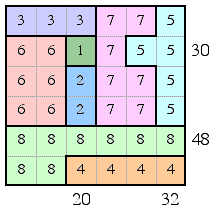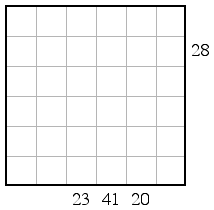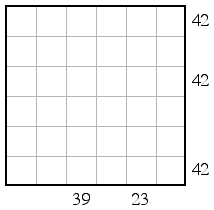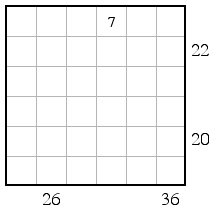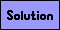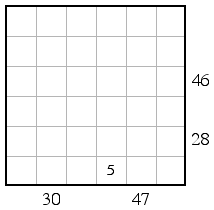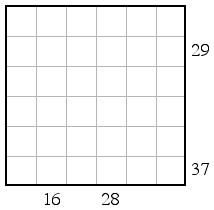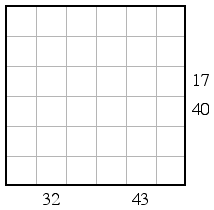Pieces of Eight Puzzles

Each square grid below contains a number from 1 to 8. There are n occurences of the digit n, and these digts are all adjacent to one another. The number on the right of a row or below a column are the sum of the digits in that row or column. Below is an example of a solved puzzle. Each puzzle below has a unique solution.